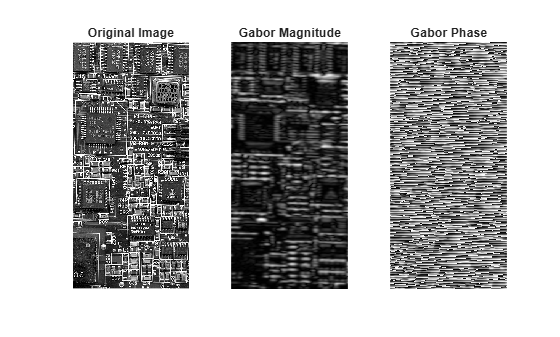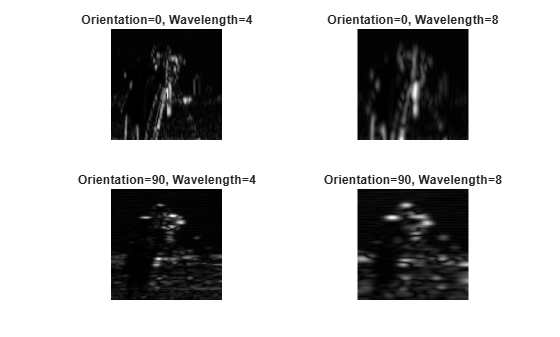imgaborfilt

Apply Gabor filter or filter bank to 2-D image

Description

example

[mag,phase] = imgaborfilt(A,wavelength,orientation) computes the magnitude and phase response of a Gabor filter for the input grayscale image A. wavelength describes the wavelength in pixels/cycle of the sinusoidal carrier. orientation is the orientation of the filter in degrees.

[mag,phase] = imgaborfilt(A,wavelength,orientation,Name,Value) applies a single Gabor filter using name-value arguments to control various aspects of filtering.

example

[mag,phase] = imgaborfilt(A,gaborbank) applies the Gabor filter bank, gaborbank, to the input image A.

Examples

collapse all

Read an image into the workspace and convert the image to grayscale.

I = im2gray(I);

Apply a Gabor filter to the image.

wavelength = 4;
orientation = 90;
[mag,phase] = imgaborfilt(I,wavelength,orientation);

Display the original image with plots of the magnitude and phase response calculated by the Gabor filter.

tiledlayout(1,3)
nexttile
imshow(I)
title('Original Image')
nexttile
imshow(mag,[])
title('Gabor Magnitude')
nexttile
imshow(phase,[])
title('Gabor Phase')Create array of Gabor filters, called a filter bank. This filter bank contains two orientations and two wavelengths.

gaborArray = gabor([4 8],[0 90]);

Apply filters to input image.

gaborMag = imgaborfilt(I,gaborArray);

Display results. The figure shows the magnitude response for each filter.

figure
subplot(2,2,1);
for p = 1:4
subplot(2,2,p)
imshow(gaborMag(:,:,p),[]);
theta = gaborArray(p).Orientation;
lambda = gaborArray(p).Wavelength;
title(sprintf('Orientation=%d, Wavelength=%d',theta,lambda));
endInput Arguments

collapse all

2-D grayscale image, specified as a numeric matrix.

Wavelength of the sinusoidal carrier, specified as a number greater than or equal to 2, in pixels/cycle. Typical values of wavelength range from 2 up to the hypotenuse length of the input image .

Orientation of the filter in degrees, specified as a numeric scalar in the range [0, 360]. The orientation is defined as the normal direction to the sinusoidal plane wave.

Gabor filter bank, specified as a gabor object or an array of gabor objects.

Name-Value Arguments

Specify optional comma-separated pairs of Name,Value arguments. Name is the argument name and Value is the corresponding value. Name must appear inside quotes. You can specify several name and value pair arguments in any order as Name1,Value1,...,NameN,ValueN.

Example: 'SpatialFrequencyBandwidth',2 specifies a spatial frequency bandwidth of two octaves

Spatial-frequency bandwidth, specified as a numeric scalar in units of octaves. The spatial-frequency bandwidth determines the cutoff of the filter response as frequency content in the input image varies from the preferred frequency, 1/lambda. Typical values for spatial-frequency bandwidth are in the range [0.5, 2.5].

Ratio of the semimajor and semiminor axes of Gaussian envelope (semiminor/semimajor), specified as a positive number. This argument controls the ellipticity of the Gaussian envelope. Typical values for spatial aspect ratio are in the range [0.23, 0.92].

Output Arguments

collapse all

Magnitude response for the Gabor filter or filter bank, returned as a numeric matrix for a single filter or a numeric array for a filter bank. The p-th plane of mag is the magnitude response for the Gabor filter of the same index, gaborbank(p).

Data Types: double

Phase response for the Gabor filter or filter bank, returned as a numeric matrix for a single filter or a numeric array for a filter bank. The p-th plane of phase is the phase response for the Gabor filter of the same index, gaborbank(p).

Data Types: double

Tips

• If the image contains Infs or NaNs, then the behavior of imgaborfilt is undefined because Gabor filtering is performed in the frequency domain.

• For all input data types other than single, imgaborfilt performs the computation in double. Input images of type single are filtered in type single. Performance optimizations may result from casting the input image to single prior to calling imgaborfilt.

 Jain, Anil K., and Farshid Farrokhnia. "Unsupervised Texture Segmentation Using Gabor Filters." Pattern Recognition 24, no. 12 (January 1991): 1167–86. https://doi.org/10.1016/0031-3203(91)90143-S.